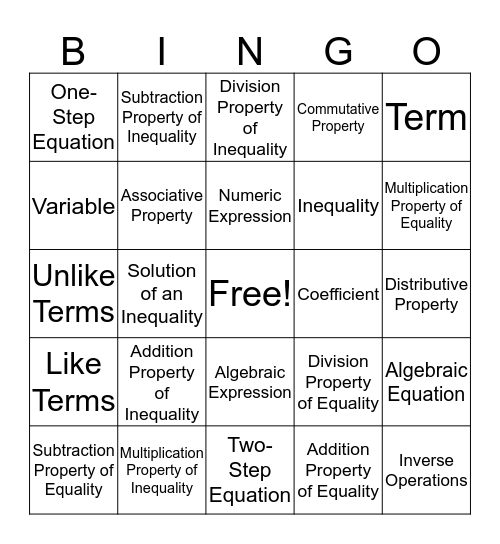# Expressions and Equations Vocabulary BingoThis bingo card has a free space and 24 words: Addition Property of Inequality, Multiplication Property of Inequality, Numeric Expression, Subtraction Property of Equality, Term, One-Step Equation, Variable, Subtraction Property of Inequality, Coefficient, Algebraic Expression, Inequality, Unlike Terms, Division Property of Equality, Algebraic Equation, Associative Property, Addition Property of Equality, Distributive Property, Division Property of Inequality, Multiplication Property of Equality, Solution of an Inequality, Inverse Operations, Like Terms, Commutative Property and Two-Step Equation.

## Play Online